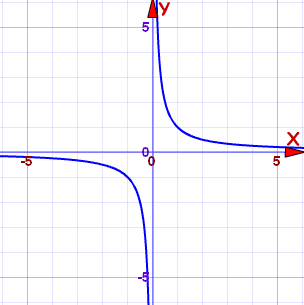# LED linear frequency change

I'm trying to get an LED to change it's frequency linearly over time. I can make it change it's frequency but it seems to change it logarithmically. I tried using the Timer.h library to change 'interval' every second or so, but no luck.. Can anyone help?

int inPin = 7; // Which port the button is connected to
int ledPin = 13; // Which port the LED is connected to
int current; // Current state of the button, 0: pressed, 1: released
float interval = 20; // Amount of milliseconds between turning on and off the LED
int counter;

void setup() {
Serial.begin(9600); // Use serial for debugging
pinMode(ledPin, OUTPUT); // Set the LED as an output
digitalWrite(inPin, HIGH); // Set the button to start at HIGH
}

void loop() {
current = digitalRead(inPin); // Reads the state of the button and defines it as 'current'

if (interval > 1) {
if (current == HIGH) { // If the button is released
interval+= 0.03; // interval goes up
}
}
if (interval > 2) {
if (current == LOW) { // If the button is pressed
interval-= 0.03; // interval goes down
}
}

//Serial.println(interval);
//Serial.println(current);
Serial.println(1000/interval);
}

digitalWrite(ledPin, HIGH);
delay (interval);
digitalWrite(ledPin, LOW);
delay(interval);
}

``````  delay (interval);
``````

delay accepts what sort of parameter?

(See what I did with code tags there?)

delay accepts milliseconds?

What data type?

``````      interval-= 0.03;                // interval goes down
``````

Are you going to see a 30 microsecond change?

A constant change is a smaller percentage of a large interval than of a small interval. Perhaps you should adjust the interval by percentage rather than by a constant.

``````  if (interval > 1) {
if (current == HIGH) {       // If the button is released
interval *= 1.03;                // interval goes up 3%
}
}
if (interval > 2) {
if (current == LOW) {        // If the button is pressed
interval *= 0.97;                // interval goes down 3%
}
}
``````

PaulS: Yes, I tried some different values and this seemed good, but it could just as well be another value

AWOL: well, interval is set to float, if that's what you mean?

Johnwasser: good idea, I'll give it a go!

Yes, That's what he means... delay() accepts ints, so the float is just converted to int. So 0,6 will just be 0. And yeah, a difference of 0,3ms will not do anything visible...

Okay thanks, but that's not really the problem, I can always change that later. It's more how the frequency changes faster the smaller interval gets

That's simply because you use delay()The smaller the delay(), the faster read the button. Because the delay() is preventing the button from being read.

Uranhjorten:
Okay thanks, but that's not really the problem, I can always change that later. It's more how the frequency changes faster the smaller interval gets

File this in the category of "DUH" for anyone that actually bothers to do math.

Period and frequency are inversely proportional to each other. Linearly changing the period will not linearly change the frequency.

Imagine this sequence:

1/10 -> 1/9 -> 1/8 -> 1/7 -> 1/6 -> etc.

The denominator is changing linearly, but does the mean the sequence (the reciprocal function f(x) = 1/x) is linear?

No it is not. Not even close.You can improve things by doing the reciprocal calculations in code, but there will still be errors due to truncation. The best way to generate a waveform with linear control of the frequency is with a numerically-controller oscillator (NCO). Unfortunately, none of the common hobbyist-friendly AVRs have such a peripheral, though there are PICs that do. You may be able to find an external chip that can do the job, such as one used for direct digital synthesis (DDS), but those are expensive and usually not in through-hole packages.